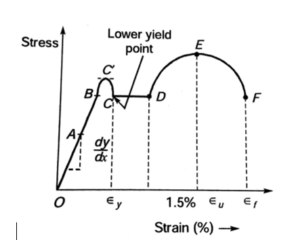Engineering Jobs   »   Govt. Job Notifcation   »   Concept Booster Mechanical

# Daily Concept Booster Mechanical Engineering: Engineering curve for mild steel for tension under static-loadingOA – Straight line (proportional region, Hooks law is valid)

OB – Elastic region

BC’ – Elasto plastic region

CD – Perfectly plastic region

DE – Strain hardening

EF – Necking region

A – Limit of proportionality

B – Elastic limit

C’ – Upper yield point

C – Lower yield point

D – Strain hardening starts

E – Ultimate stress

F – Fracture point

### Limit of proportionality

it is the point at which the stress-strain curve ceases to be a straight line.

### Hooks law

When a material behaves elastically and exhibits a linear relationship between stress and strain, it’s called linearly elastic. In this stress (σ) is directly proportional to strain (ϵ)Where,         σ = Stress,  ϵ=Strain

E = Young’s Modulus of elasticity

### Elastic limit

It is the point in the stress-strain curve up to which the materials remain elastic. Up to this point, there is no permanent deformation after the removal of load.

### Plastic Range

It is the region of the stress-strain curve between the elastic limit and point of rupture.

### Yield point

This point is just beyond the elastic limit, at which the specimen undergoes an appreciable increase in length without further increase in the load.### Rupture strength

It is the stress corresponding to the failure point ‘F’ of the stress-strain curve.

### Proof stress

It is the stress necessary to cause a permanent extension equal to a defined percentage of the gauge length.

The slope of OA = Modulus of elasticity (Young’s Modulus)  It is the constant of proportionality which is defined as the intensity of stress that causes unit strain.
Plastic strain is 10 to 15 times elastic strain

• Fracture strain(ϵf)depends on the percentage of carbon in the steel.
• When carbon percentage increases then fracture strain decreases and yield stress increases.

Boost your preparation with the all-new Concept Booster Series by Adda247 Engineering Jobs, this series aims to target all the important engineering exams such as SSC Je 2022, GATE 2022, ESE 2022, and many more. Please reach out to us with your feedback in the comment section.Sharing is caring!